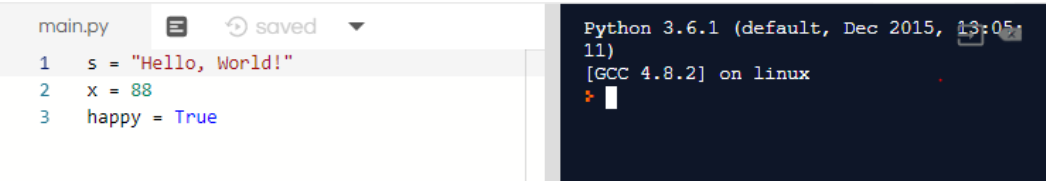# Variables

Variables are simply names that we can give to values such as strings, numbers, and booleans. Here’s how to make a variable named s. We say s is a string that has the value "Hello, World!". Can you describe the following variables?

s = "Hello, World!"
x = 88
happy = True


Press run.Note that variables are not printed out to the console. Instead, the variable simply saves the string, number, or boolean into the computer’s memory. We can use these variables in other statements. For example, the following code would print "Hello Nuevo Foundation" to the console:

str1 = "Hello"
str2 = "Nuevo Foundation"
print(str1 + " " + str2)


You can also do the following to print strings together while adding spaces in between the words.

str1 = "Hello"
str2 = "Nuevo Foundation"
print(str1, str2)


### Challenge

• Create two new variables: A variable named comp that stores the string "Computer".
• A variable named five that stores the number 5.

Next, use the variables and what you learned in the previous activities to print out the following to the console. You must use the variables!

Computer
5
ComputerComputer
10
ComputerComputerComputer
15